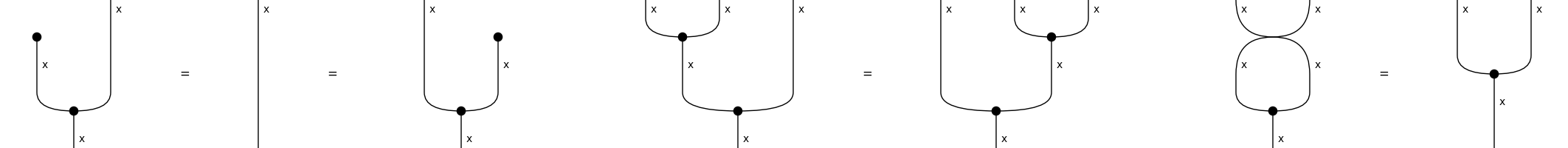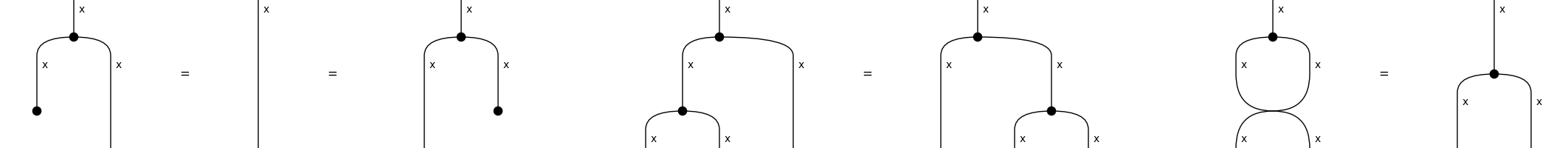# markov#

The free Markov category, i.e. a semicartesian category with a supply of commutative comonoid, see Fritz and Liang [FL23].

## Summary#

 `Diagram` A Markov diagram is a symmetric diagram with `Copy` boxes. `Box` A Markov box is a symmetric box in a Markov diagram. `Swap` Symmetric swap in a Markov diagram. `Copy` The copy of an atomic type `x` some `n` number of times. `Category` A Markov category is a symmetric category with a method `copy`. `Functor` A Markov functor is a symmetric functor that preserves copies.

## Axioms#

```>>> from discopy.drawing import Equation
>>> Diagram.use_hypergraph_equality = True
>>> x = Ty('x')
```
```>>> copy, merge = Copy(x), Merge(x)
>>> unit, delete = Merge(x, n=0), Copy(x, n=0)
```
• Commutative monoid:

```>>> unitality = Equation(unit @ x >> merge, Id(x), x @ unit >> merge)
>>> associativity = Equation(merge @ x >> merge, x @ merge >> merge)
>>> commutativity = Equation(Swap(x, x) >> merge, merge)
>>> assert unitality and associativity and commutativity
>>> Equation(unitality, associativity, commutativity, symbol='').draw(
...     path="docs/_static/frobenius/monoid.png")
```• Cocommutative comonoid:

```>>> counitality = Equation(copy >> delete @ x, Id(x), copy >> x @ delete)
>>> coassociativity = Equation(copy >> copy @ x, copy >> x @ copy)
>>> cocommutativity = Equation(copy >> Swap(x, x), copy)
>>> assert counitality and coassociativity and cocommutativity
>>> Equation(counitality, coassociativity, cocommutativity, symbol='').draw(
...     path="docs/_static/frobenius/comonoid.png")
```• Coherence:

```>>> assert Diagram.copy(x @ x, n=0) == delete @ delete
>>> assert Diagram.copy(x @ x)\
...     == copy @ copy >> x @ Swap(x, x) @ x
>>> assert Diagram.merge(x @ x, n=0) == unit @ unit
>>> assert Diagram.merge(x @ x)\
...     == x @ Swap(x, x) @ x >> merge @ merge
```
```>>> Diagram.use_hypergraph_equality = False
```

Note

Equality of Markov diagrams is computed by translation to hypergraph. Both copy and merge boxes are translated to spiders, thus when they appear in the same diagram they automatically satisfy the `frobenius` axioms.